## 基于MATLAB的图像处理笔记

84
0
2020年11月13日 08时53分## 一、基础操作

1.1 基本函数Mean():
M = mean(A)Mat2gray():
I =mat2gray(A, [amin amax])

Imcrop():
I2 = imcrop(I,rect)

1.图像相加可以减少噪声，也可以用于增加曝光度（high dynamic range image）
2.图像相减可以用于运动检测
3.在图像显示的时候，要先调成uint8的类型，否则无法正常显示。注意，对图像的操作大部分基于double格式的。

1.2 色彩空间YCbCr其中Y是指亮度分量，Cb指蓝色色度分量，而Cr指红色色度分量。人的肉眼对视频的Y分量更敏感，因此在通过对色度分量进行子采样来减 少色度分量后，肉眼将察觉不到的图像质量的变化。

J=rgb2ycbcr(I);
K=J(:,:,1); %1是Y，2是Cb，3是Cr

1.3 灰度变换

imhist( i ,n)：

J=histeq（I，n）:
I为输入的原图像，J为直方图均衡化后得到的图像，n为均衡化后的灰度级数，默认值为641.4 色彩平衡算法

a.GrayWorld 算法：

grayworld解释：grayworld代码：

function y=GrayWorld(Image)
%RGB图像为n*m*3的数组，表示尺寸为n*m个像素，其中每个像素由RGB三个分量构成。(:,:,1)表示遍历xy，提取红色分量
r=Image(:,:,1);
g=Image(:,:,2);
b=Image(:,:,3);
%取平均值，因为是二维的，故用两次mean函数
avgR = mean(mean(r));
avgG = mean(mean(g));
avgB = mean(mean(b));
avgRGB = [avgR avgG avgB];
grayValue = (avgR + avgG + avgB)/3
scaleValue = grayValue./avgRGB;

newI(:,:,1) = scaleValue(1) * r;
newI(:,:,2) = scaleValue(2) * g;
newI(:,:,3) = scaleValue(3) * b;

y=uint8(newI);



b. ScaleByMax算法

scaleByMax解释：scaleByMax代码;

function y=ScaleByMax(img)
R = img(:,:,1);
G = img(:,:,2);
B = img(:,:,3);
Rmax=max(max(R));
Gmax=max(max(G));
Bmax=max(max(B));

Cmax=min([Rmax,Gmax,Bmax]);

% calculate the pix,which larger than Cmax
Nr=sum(R(:)>=Cmax);
Ng=sum(G(:)>=Cmax);
Nb=sum(B(:)>=Cmax);

% find the largest N for Nr Ng Nb
Nmax=max([Nr,Ng,Nb]);

% for each of the find the number of element which is larger than Rmax;
for pix=Rmax:-1:0  % R
Lr=R>=pix;
nr=sum(Lr(:));
if nr>=Nmax
Rth=pix;
break
end
end

for pix=Gmax:-1:0   % G
Lr=G>=pix;
nr=sum(Lr(:));
if nr>=Nmax
Gth=pix;
break
end
end

for pix=Bmax:-1:0 % B
Lr=B>=pix;
nr=sum(Lr(:));
if nr>=Nmax
Bth=pix;
break
end
end
result(:,:,1) = Cmax/Rth*R;
result(:,:,2) = Cmax/Gth*G;
result(:,:,3) = Cmax/Bth*B;
y = result;



## 二、空间域操作

2.1 二维卷积和滤波

Conv2(): 求二维卷积
C = conv2(A,B,shape)shape用于处理边缘，有’same’’full’’valid’三种之分，以same为例：conv2(A,B,’same’)产生的结果与A的大小相同，因此，用‘same’时把卷积核放在B位置，否则出来图像和卷积核一样大。

Imfilter(): 线性滤波
B = imfilter(A,h)

medfilt2(): 二维的中值滤波
B = medfilt2(A, [m n])

Imnoise(): 添加噪声
J = imnoise(I,type,parameters)

2.2 滤波算子

fspecial(): 函数用于产生卷积核，用于特定的滤波
h=fspecial(type,parameters)

type的类型有：
1、‘average’
h = fspecial(‘average’, hsize)

Eg:
0.1111 0.1111 0.1111
0.1111 0.1111 0.1111
0.1111 0.1111 0.1111
2、 ‘disk’
circular averaging filter

3、‘gaussian’
Gaussian lowpass filter
h = fspecial(‘gaussian’, hsize, sigma)

Eg:
0.0000 0.0000 0.0000
0.0000 1.0000 0.0000
0.0000 0.0000 0.0000
4、‘laplacian’ filter approximating the 2-D Laplacian operator
h = fspecial(‘laplacian’, alpha)

Eg:
0.3333 0.3333 0.3333
0.3333 -2.6667 0.3333
0.3333 0.3333 0.3333
5、‘log’
Laplacian of Gaussian filter
h = fspecial(‘log’, hsize, sigma)

6、‘motion’
h = fspecial(‘motion’, len, theta)
motion filter为运动模糊算子，有两个参数，表示摄像物体逆时针方向以theta角度运动了len个像素，len的默认值为9，theta的默认值为0；
7、‘prewitt’
Prewitt horizontal edge-emphasizing filter

(注：若卷积核用于突出水平边缘特征（sober,prewitt），那么转置后便可突出垂直边缘特征，转置用transpose函数)
8、‘sobel’
Sobel horizontal edge-emphasizing filter

9、‘unsharp’
unsharp contrast enhancement filter

2.3 非局部均值去噪滤波：Non-Local Meansfunction [output]=simple_nlm(input,t,f,h1,h2,selfsim)
[m,n]=size(input);
pixels = input(:);
s = m*n;
psize = 2*f+1;
nsize = 2*t+1;
% Compute patches
filter = fspecial('gaussian',psize,h1);
patches = repmat(sqrt(filter(:))',[s 1]) .* im2col(padInput, [psize psize], 'sliding')';
% Compute list of edges (pixel pairs within the same search window)
indexes = reshape(1:s, m, n);
neighbors = im2col(padIndexes, [nsize, nsize], 'sliding');
TT = repmat(1:s, [nsize^2 1]);
edges = [TT(:) neighbors(:)];
RR = find(TT(:) >= neighbors(:));
edges(RR, :) = [];
% Compute weight matrix (using weighted Euclidean distance)
diff = patches(edges(:,1), :) - patches(edges(:,2), :);
V = exp(-sum(diff.*diff,2)/h2^2);
W = sparse(edges(:,1), edges(:,2), V, s, s);
% Make matrix symetric and set diagonal elements
if selfsim > 0
W = W + W' + selfsim*speye(s);
else
maxv = max(W,[],2);
W = W + W' + spdiags(maxv, 0, s, s);
end
% Normalize weights
W = spdiags(1./sum(W,2), 0, s, s)*W;
% Compute denoised image
output = W*pixels;
output = reshape(output, m , n);



## 三、频域操作

3.1 离散傅里叶变换：DFT

fft2(): 二维傅里叶变换
Y = fft2(X,m,n)
pads X with zeros to create an m-by-n array before doing the transform. The result is m-by-n.
Y=fft2(X)
returns the two-dimensional discrete Fourier transform (DFT) of X, if size(X) = [100 100 3], then fft2 computes the DFT of X(:,:,1), X(:,:,2) and X(:,:,3)3.1.1高通滤波3.1.2 低通滤波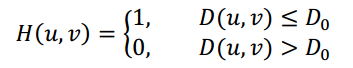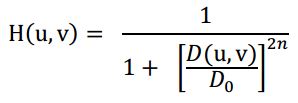Imshow时检查矩阵是否为double类型，要转化为uint8才能正常输出。

3.2 离散余弦变换：DCT

DCT变换公式：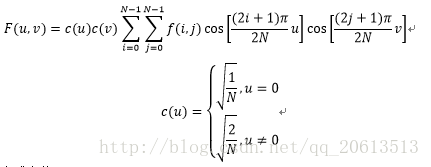DCT优势：
1.The most important reason is much reduced sensitivity to boundaries of finite sequences（DFT变换没有考虑到子图之间的边界，波形不连续容易导致Gibbs phenomenon；而DCT得到子图间的波形则是连续的。）
2 Resulting Compaction（可压缩）
3 No complex numbers（无复数，因只取实部进行变换，实偶函数傅里叶变换也是实偶函数）
4 Number of coefficients is equal to number of pixels（变换后一个像素对应一个值）
5 DCT coefficients occur at every half cycle so Nyquist can be stated as number of coefficients should not exceed number of
sample points

3.2.1 DCT域滤波Meshgrid():
[X,Y] = meshgrid(x,y)
[X,Y,Z] = meshgrid(x,y,z)

Surface():
surface(X,Y,Z)

View():
view(az,el)Surf():
surf(X,Y,Z)

3.2.2维纳滤波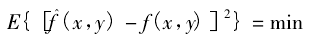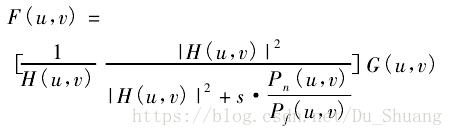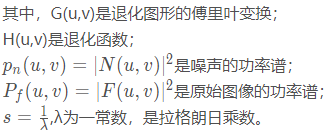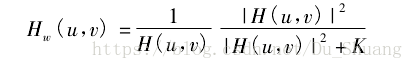MATLAB对维纳滤波算法的解释：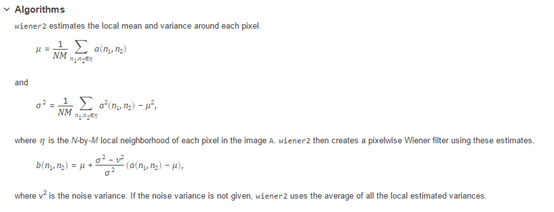sigma = d_im1_Y(100:end,100:end).*d_im1_Y(100:end,100:end);%d_im1_Y为图片im1的Y分量的dct，对dct变换矩阵的右下部分进行滤波，
NoiseVariance = mean(mean(sigma));%算均值，评估噪声强度，需要多次试验得出最佳系数
beta = 4.0;%与噪声移除有关的系数，越高噪声滤除越多，但图片也越模糊
NoiseVariance = beta*NoiseVariance;
SignalVariance = d_im1_Y.*d_im1_Y + 0.001;%
WienerFilter = 1 + (NoiseVariance./SignalVariance);
WienerFilter = 1./WienerFilter;
FilteredImageDCT = d_im1_Y.*WienerFilter;
FilteredImage = idct2(FilteredImageDCT);
imo = uint8(FilteredImage);
imshow(imo);



wiener2():
J = wiener2(I,[m n],noise)

[J,noise] = wiener2(I,[m n])

J=deconvwnr（I,PSF,NSR）：维纳滤波图像复原
PSF为点扩散函数，可以由fspecial函数创建（比如PSF = fspecial(‘motion’);）， PSF与原始图像进行卷积，可得到退化（模糊）的图像。用于复原由于PSF以及可能的加性噪声卷积退化的图像I，该算法利用图像和噪声的相关矩阵，从估计图像与真实图像之间的最小均方误差。在没有噪声的情况下，维纳滤波器退化成理想的逆滤波器。NSR是信噪功率比，NSR可以是标量，或者是和图像I一样大小尺寸的数组，NSR的默认值为0。3.2.3 DCT域对比增强

im = imread('moon.tif');
imd = dct2(im);
dc = imd(1,1);
imd=imd*1.4;%乘一个比例系数，越大对比度越明显
imd(1,1)=dc;%保持直流分量不变
im2=idct2(imd);
%%%%%%%%%%%%%%%%%%减一个比例系数x
a= min(min(im2))
b= max(max(im2))-255;
x = max(a,b)

figure
imshow(im);
figure
imshow(uint8(im2-x));



3.2.4 DCT域有损压缩：JPEG

1、将原始图像分为 8 * 8 的小块, 每个 block 里有 64 个像素。

2、 将图像中每个 8 * 8 的 block 进行 DCT 变换。8 * 8 的图象经过 DCT 变换后,其低频分量都集中在左上角,高频分量分布在右下角。由于该低频分量包含了图象的主要信息(如亮度),而高频与之相比,就不那么重要了,所以我们可以忽略高频分量。

3、 利用量化表抑制高频变量。量化操作,就是将某一个值除以量化表中对应的值。由 于量化表左上角的值较小,右上角的值较大,这样就起到了保持低频分量,抑制高 频分量的目的。舍弃高频系数（AC系数），保留低频信息（DC系数）。高频系数一般保存的是图像的边界、纹理信息，低频信息主要是保存的图像中平坦区域信息。图像的低频和高频，高频区域指的是空域图像中突变程度大的区域（比如目标边界区域），通常的纹理丰富区域。

4.压缩时候将彩色图像的颜色空间由 RGB 转化为 YUV 格式。其中 Y 分量代表了亮度信息,UV 分量代表了色差信息。相比而言,Y 分量更重要一些。 我们可以对 Y 采用细量化,对 UV 采用粗量化,可进一步提高压缩比。所以量化表 通常有两张,一张是针对 Y 的标准亮度量化表;一张是针对 UV 的标准色彩量化表。

5.经过量化之后右下角大部分数据变成了 0,左上角为非零数据。这时使用 Z 字型(如 图所示)的顺序来重新排列数据生成一个整数数组,这样 0 就位于数组都后端。找到数组最后一个非零元素,将其后的数据都舍弃,并加上结束标志。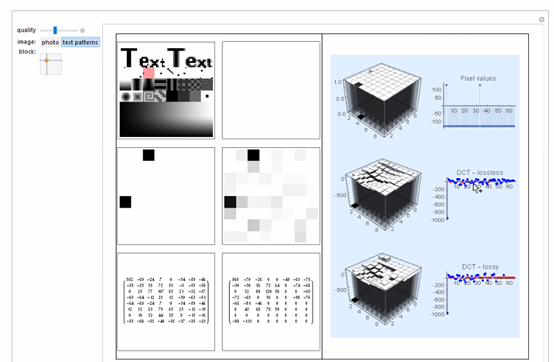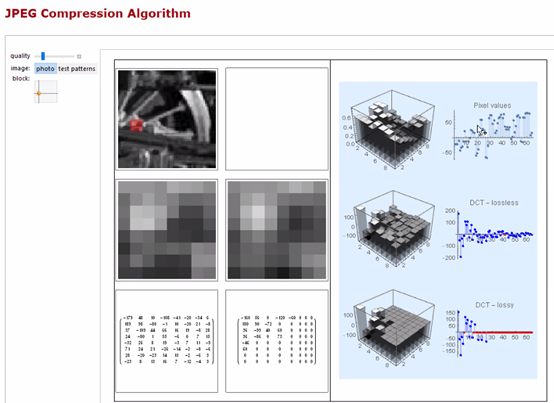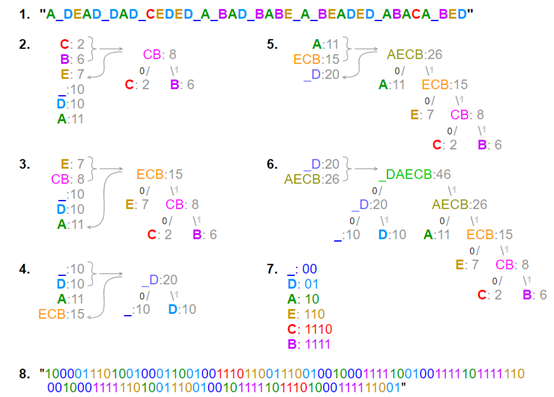## 4.图像分割

4.1 基于导数的边缘识别

1.导数，连续函数上某点斜率，导数越大表示变化率越大，变化率越大的地方就越是“边缘”，但是在计算机中不常用，因为在斜率90度的地方，导数无穷大，计算机很难表示这些无穷大的东西。
2.微分与差分，连续函数上x变化了dx，导致y变化了dy，dy值越大表示变化的越大，那么计算整幅图像的微分，dy的大小就是边缘的强弱了。差分与微分的离散形式。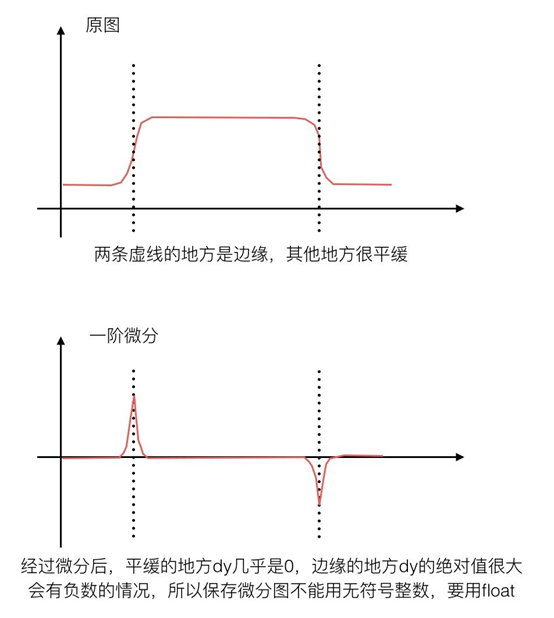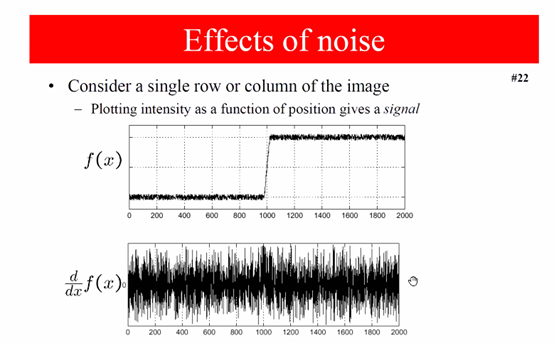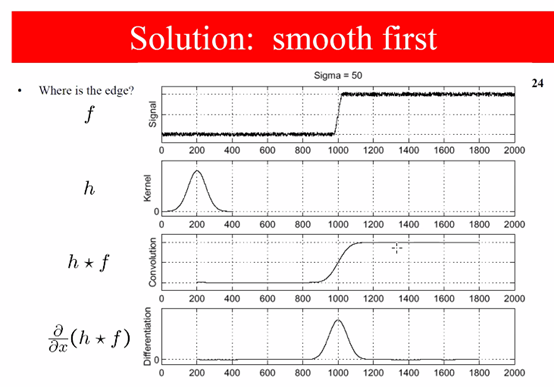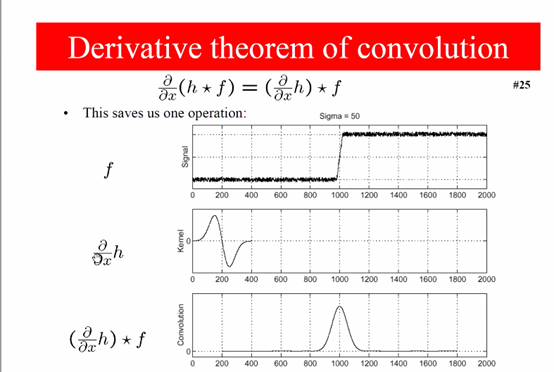4.1.1 基于一阶导数的边缘检测

Sobel算子（prewitt算子类似）：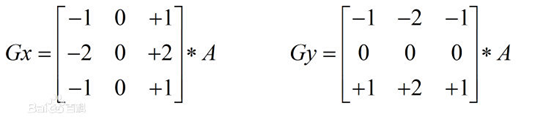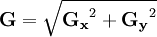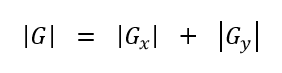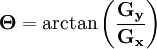sobel算子原理：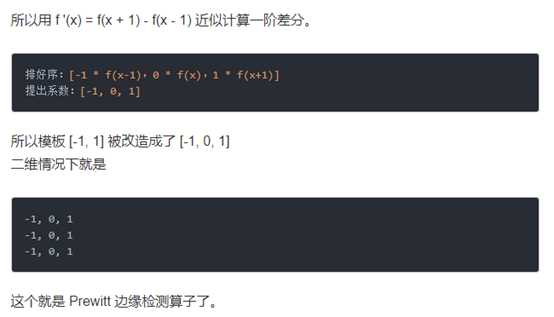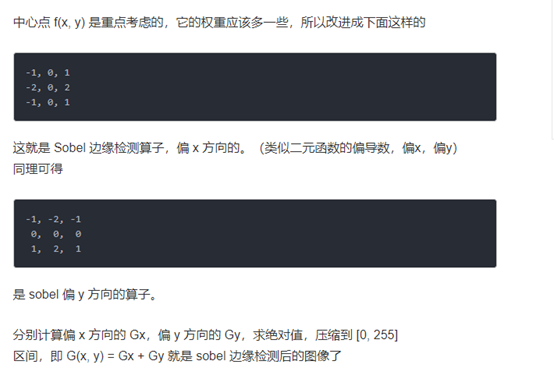Roberts算子：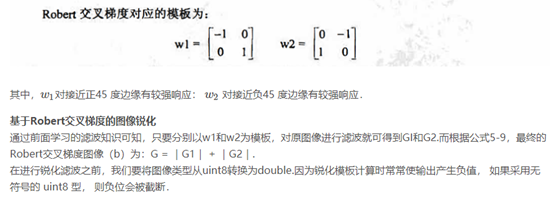Roberts算子定位精度高，但受噪声影响大。对于陡峭边缘的低噪声图像效果最好。

4.1.2 基于二阶导数的边缘检测

Laplace算子：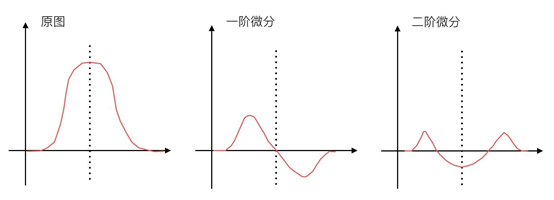Laplace算子实现原理：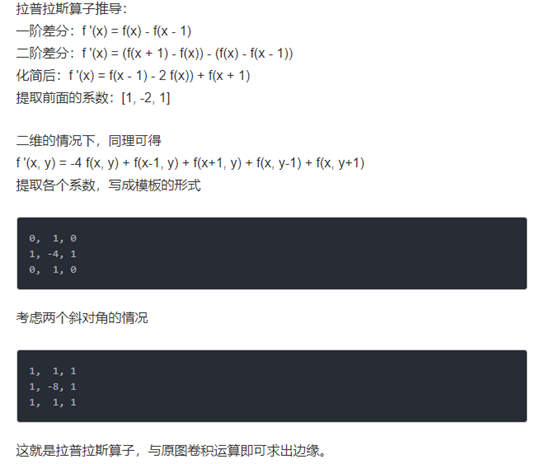4.2 Canny算子及原理

Canny边缘检测算法可以分为5步：
1.对图片（灰度图）进行高斯平滑滤波，去除噪声
2.计算梯度强度和方向
3. 非极大值抑制（non-maximum suppression），使边缘细化
4.双阈值检测（double thresholding）
5. 抑制孤立低阈值点

1.灰度图与高斯平滑滤波2.计算梯度，x方向，y方向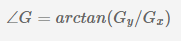得到梯度的方向
![在这里插入图片描述](https://img-blog.csdnimg.cn/201905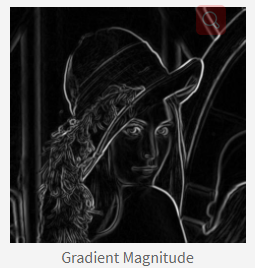3.非极大值抑制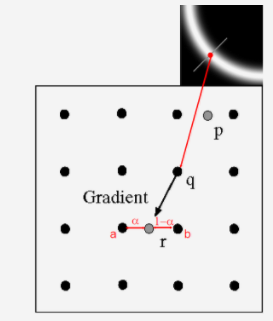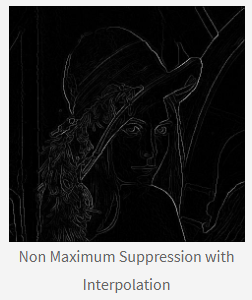4.双阈值检测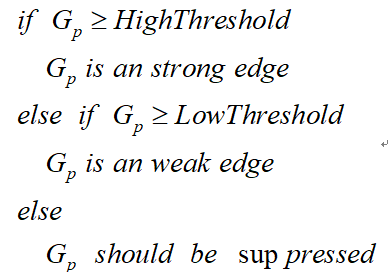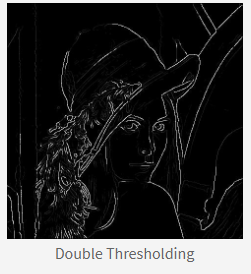5. 抑制孤立低阈值点和边缘连接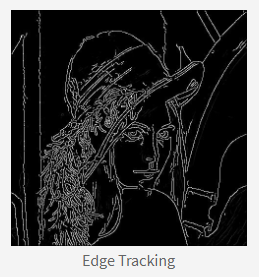Edge()
[g,t]=edge(I,’method’,parameters)
g为二值图像，t为阈值，I是输入图像，method是边缘检测算子，parameters是设置的参数，parameters省略时系统将自动设置参数。以下对各算子分别说明：
BW = edge(I,‘Sobel’,threshold,direction,options)

BW = edge(I,‘Canny’,threshold,sigma)
Threshold为阈值，若为两元素向量，则为[low high], 其中0 < low < high < 1；若为一个元素，则第一个元素为高阈值，低阈值为其0.5倍。Sigma为高斯滤波器的标准差，缺省值为1。’log’算子类似，不赘述。

4.4Hough变换
Hough变换是图像处理中从图像中识别几何形状的基本方法之一。Hough变换的基本原理在于利用点与线的对偶性，将原始图像空间的给定的曲线通过曲线表达形式变为参数空间的一个点。这样就把原始图像中给定曲线的检测问题转化为寻找参数空间中的峰值问题。也即把检测整体特性转化为检测局部特性。比如直线、椭圆、圆、弧线等。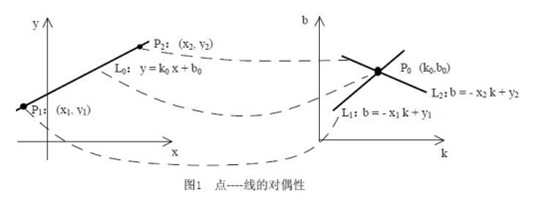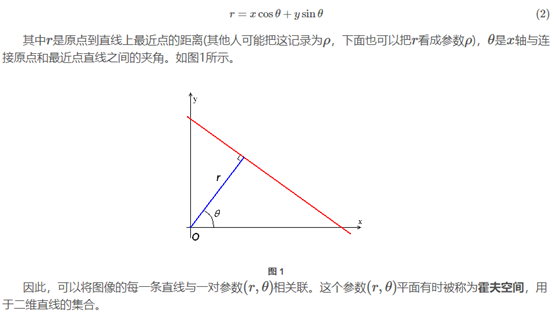Hough变换后，一个点映射到hough空间得：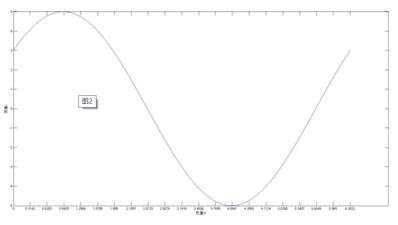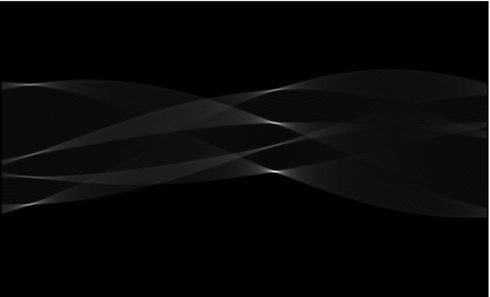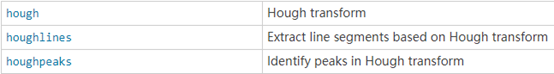Hough():
[H,theta,rho] = hough(BW)

Houghpeaks():
peaks = houghpeaks(H,numpeaks)
H为霍夫变换后的矩阵，numpeaks是找出峰值点的个数，默认1.返回peaks是各点坐标的集合，Q*2的矩阵，Q为峰值点个数。

Houghlines():
lines = houghlines(BW,theta,rho,peaks)

figure, imshow(image), hold on

max_len = 0;
for k = 1:length(lines)
xy = [lines(k).point1; lines(k).point2];
plot(xy(:,1),xy(:,2),'LineWidth',2,'Color','green');

% Plot beginnings and ends of lines
plot(xy(1,1),xy(1,2),'x','LineWidth',2,'Color','yellow');
plot(xy(2,1),xy(2,2),'x','LineWidth',2,'Color','red');

% Determine the endpoints of the longest line segment
len = norm(lines(k).point1 - lines(k).point2);
if ( len > max_len)
max_len = len;
xy_long = xy;
end
end
% highlight the longest line segment
plot(xy_long(:,1),xy_long(:,2),'LineWidth',2,'Color','red');